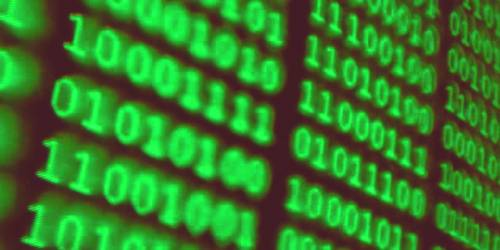Technology

# Algorithm and its Binary SearchingAn algorithm is a well-defined list of steps for solving a particular problem. One major purpose of this text is to develop an efficient algorithm for the processing of our data. The time and space it uses are two major measures of the efficiency of an algorithm. It is a procedure to be followed in calculations or other problem-solving operations, mainly by a computer. It is usually used for data processing, calculation, and other related computer and mathematical operations.

An algorithm of binary searching – Binary search is the most popular Search algorithm. Binary search works only on a sorted set of elements.

Binary search algorithm –

1. [Initialize segment variables]

Set BEG: = LB, END: = UB and MID = INT [(BEG+END)/2]

1. Repeat steps 3 and 4 while BEG ≤ END and DATA [ ] ≠ ITEM.
2. If Item < DATA [MID], then:

Set END: = MID – 1

Else: Set BEG: = MID + 1

[End of If Structure]

1. Set MID: = INT [(BEG + END) / 2]

[End of Step 2 loop].

1. If DATA [MID] = ITEM, then

Set LOC: = MID

Else: Set LOC: NULL

[End of if Structure]

1. Exit.

The complexity of Binary search algorithm –

The complexity is measured by the number f(n) of comparisons to locate ITEM in DATA where DATA contains n elements. In a binary search algorithm, each comparison reduces the sample size in half. Hence we required at most f(n) comparisons to locate ITEM where 2f(n) > n, or equivalently f(n) = [log2n] + 1.

That is the running time, for the worst case is approximately equal to log2. One can also show that the running time for the average case is approximately equal to the running time for the worst case.